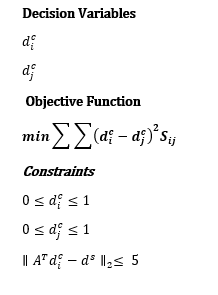# Writing an objective function with summation over two decision variables in CVXI am trying to implement the model posted above in CVX. How can I write this formulation in Matlab?

Do you really just have a single vector decision variable, of length, n? If so, your last constraint looks a little strange, as the variable is indexed by i even though it looks like a matrix-vector multiplication.

If there is a single decision variable of length n, you could program the objective function by brute force, presuming all elements of S are non-negative.

``````cvx_begin
variable d(n)
Obj = 0;
for i=1:n, for j=1:n, Obj = Obj + (d(i)-d(j))^2*S(i,j); end, end
minimize(Obj)
``````

Then add your constraints. Perhaps you can vectorize this code, but that is not necessary.

If this is not correct, you need to make clear what your optimization problem is, including all dimensions of variables and constraints…

Thanks for replying Mark it does seem helpful. Let me try and elaborate the problem for your as well.

So is this what you want? Other than the penalty term in the objective, which is just a constant which doesn’t affect the argmin,.this is what I was suggesting above, in which I removed an extraneous subscript i in the last constraint.

``````cvx_begin
variable d(n)
Obj = P*a;
for i=1:n, for j=1:n, Obj = Obj + (d(i)-d(j))^2*S(i,j); end, end
minimize(Obj)
0 <= d <= 1
norm(A' * d - d_school_need) <= a
cvx_end
``````

If not, hopefully you can fine tune to what you want.

yeah, this is pretty much what I was looking for but the only problem with this is that obj is computed iteratively, which is very slow in execution. Can we write the objective function better way, maybe by using some matrix argument?

As I wrote above, I leave any possible vectorization as an exercise for you.

I think you can make the current version a little faster by changing to
`for j=setdiff(1:n,i)`
so that Obj terms equal to zero are skipped.

Thanks, Mark.

I did try this before but it only improves the solution time by one second.

I think they key here to reduce the solution time is writing the obj as matrix argument. So, far I haven’t found any function in CVX that can help me do that? Do u think writing my own CVX function is required here?

I didn’t figure out a way to vectorize or matrixize the objective. I don’t know whether anyone else can.

Yeah, that’s what I have been working on since last night but I couldn’t figure out yet.

Thanks for your help, I’ll share if I make some progress on that end.

Also, do you think I can use some other software etc for this problem?